﻿ 基于多目标优化的虚拟机放置方法文章快速检索 高级检索
 重庆邮电大学学报(自然科学版)2020, Vol. 32Issue (3): 356-367  DOI: 10.3979/j.issn.1673-825X.2020.03.0040

### 引用本文LI Shuangli, LI Zhihua, YU Xinrong. Virtual machine placement method based on multi-objective optimization[J]. Journal of Chongqing University of Posts and Telecommunications (Natural Science Edition), 2020, 32(3): 356-367.   DOI: 10.3979/j.issn.1673-825X.2020.03.004.### Foundation item

The Innovations Fund for the Integration of Industry, Education and Research of Jiangsu Province under Grant (BY2013015-23)

.

### 文章历史

1. 江南大学 物联网工程学院, 江苏 无锡, 214122;
2. 江南大学 物联网应用技术教育部工程研究中心, 江苏 无锡, 214122

Virtual machine placement method based on multi-objective optimization
1. School of Internet of Things Engineering, Jiangnan University, Wuxi 214122, P. R. China;
2. Engineering Research Center of IoT Technology Application Ministry of Education, Wuxi 214122, P. R. China
Abstract: In the virtual machine placement problem, the traditional heuristic methods are not entirely applicable to the complex cloud computing environment, and the researches using intelligent algorithms lack the consideration of time overhead. To solve the above problems, a Memetic algorithm-based virtual machine placement (MAVMP) method is proposed. Firstly, The MAVMP method establishes a multi-objective optimization model for minimizing energy consumption, minimizing the service-level agreement violation times per active host (SLATAH) and maximizing resource utilization according to the operation situation of cloud data centers. Secondly, on the basis of resource requests, virtual machines are classified, improving the Memetic algorithm. Finally, the improved Memetic algorithm is used to solve the multi-objective optimization model, and then obtain the virtual machine placement plan. The results of simulation test show that the simulation data center using the MAVMP method to place virtual machines has good performances in energy consumption, resource utilization and service quality. Moreover, in contrast to the existing intelligent algorithm-based virtual machine placement method, the calculation time of the MAVMP method decreases sharply.
Keywords: cloud computing    virtual machine placement    multi-objective optimization    multiple resources    Memetic algorithm
0 引言

1 虚拟机放置

2 系统模型及问题描述

2.1 数据中心描述

2.2 能耗估算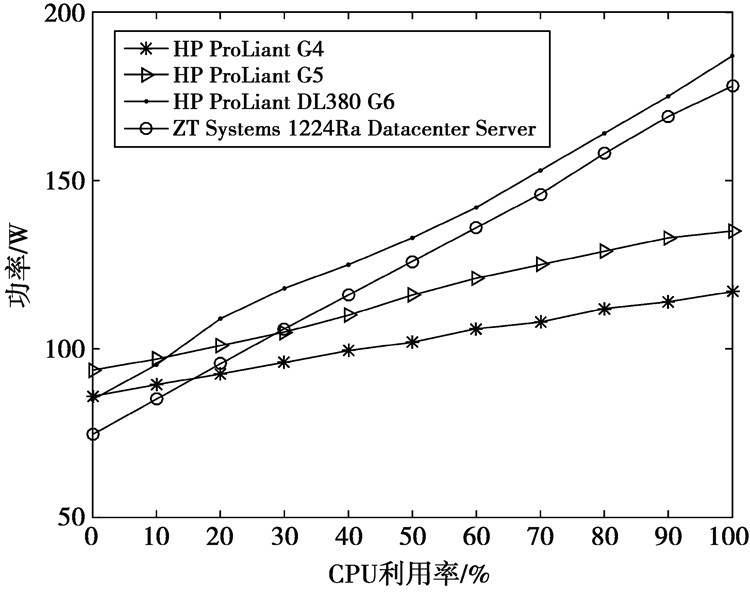图 1 CPU利用率与功率关系 Fig.1 Relation between CPU utilization and host power
 ${{f_{{\rm{ power }}}}({h_i}) = \int_0^T {{P_d}} + k \times (u - {U_d}){\rm{d}}t}$ (1)
 ${k = \frac{{{P_u} - {P_d}}}{{{U_u} - {U_d}}}}$ (2)
 ${u = f(t)}$ (3)

(1)—(3)式中：u表示物理主机的利用率；Pd表示利用率u所处区间下限的功率；Pu表示利用率u所处区间上限的功率；Ud表示负载区间下限；Uu表示负载区间上限，负载u随着时间t变化而改变。

2.3 物理主机服务等级协议违例时间估算

 ${f_{{\rm{ slatah }}}}({h_i}) = \frac{{{T_{si}}({D^r}({h_i}) > {C^r}({h_i}))}}{{{T_{ai}}}}, r{\kern 1pt} {\kern 1pt} \in {\kern 1pt} {\kern 1pt} {\kern 1pt} RS$ (4)

(4) 式中：Tsi是物理主机i出现服务等级协议违例的时间，即物理主机资源请求大于其资源总量的时间；Tai是物理主机i处于活动状态的总时间。

2.4 数据中心资源利用率估算

 ${f_{{\rm{ utilization }}}}({h_i}) = \frac{1}{N}\sum\limits_{r{\kern 1pt} \in {\kern 1pt} {\kern 1pt} RS} {\frac{{{D^r}({h_i})}}{{{C^r}({h_i})}}} , r{\kern 1pt} {\kern 1pt} \in {\kern 1pt} {\kern 1pt} {\kern 1pt} RS$ (5)

(5) 式中，N代表物理主机资源种类的数量，本文中N=3。(5)式利用物理主机hi的实际资源请求和资源总量求得该物理主机所有资源的平均利用率。

2.5 虚拟机放置优化模型

 ${{\rm{目标}}:{\rm{min}}{F_{{\rm{ power }}}}(H, X)}$ (6)
 ${{\rm{min}}{F_{{\rm{ slatah }}}}(H, X)}$ (7)
 ${{\rm{max}}{F_{{\rm{ utilization }}}}(H, X)}$ (8)
 $(6) - (8){\rm{ 中 }}:{F_{{\rm{ power }}}}(H, X) = \sum\limits_{i = 1}^n {{f_{{\rm{ power }}}}} ({h_i})$ (9)
 ${F_{{\rm{ slatah }}}}(H, X) = \frac{1}{n}\sum\limits_{i = 1}^n {{f_{{\rm{ slatah }}}}} ({h_i})$ (10)
 ${F_{{\rm{ utilization }}}}(H, X) = \frac{1}{n}\sum\limits_{i = 1}^n {{f_{{\rm{ utilizition }}}}} ({h_i})$ (11)
 ${\rm{约束}}:\sum\limits_{i = 1}^n {{x_{i, j}}} = 1{\kern 1pt} {\kern 1pt} {\kern 1pt} {\kern 1pt} {\kern 1pt} {\kern 1pt} {\kern 1pt} {\kern 1pt} {\kern 1pt} {\kern 1pt} {\kern 1pt} {\kern 1pt} {\kern 1pt} {\kern 1pt} {\kern 1pt} {\kern 1pt} {\kern 1pt} {\kern 1pt} j{\kern 1pt} {\kern 1pt} \in {\kern 1pt} {\kern 1pt} {\kern 1pt} [1, \cdots , m]$ (12)
 $\begin{array}{*{20}{l}} {\sum\limits_{j = 1}^m {{C^r}} (v{m_j}) \times {U^r}(v{m_j}) \times {x_{i, j}} < {C^r}({h_i})}\\ {i{\kern 1pt} {\kern 1pt} {\kern 1pt} \in {\kern 1pt} {\kern 1pt} {\kern 1pt} [1, \cdots , n], r{\kern 1pt} {\kern 1pt} {\kern 1pt} \in {\kern 1pt} {\kern 1pt} {\kern 1pt} RS} \end{array}$ (13)

(6)—(8)式分别代表虚拟机按照矩阵X放置在物理主机集合H上，估算得到的数据中心能耗最低，服务等级协议违例率(service level agreement violation, SLAV)最低以及资源利用率最高几种情况。(9)—(11)式利用(3)—(5)式计算数据中心的能耗、服务等级协议违例率和平均资源利用率。(12)式约束一台虚拟机只能放置在一台物理主机上。(13)式约束放置在一台物理主机上的虚拟机请求资源的总和应该小于物理主机的资源总量。

3 模型求解

Memetic算法来源于文化算法与基因算法的融合。近年来，许多研究[18-21]表明Memetic算法在多目标优化问题上有着良好的表现。但虚拟机放置问题具有多资源的特性，传统Memetic算法无法同时优化多种资源配置，目前针对多资源虚拟机调度的研究还比较少。

1) 初始化待放置虚拟机集合、物理主机集合，获取虚拟机的资源请求记录和物理主机的资源利用记录。

2) 为虚拟机和物理主机进行编码，并初始化种群，创建Pareto集合。

3) 对种群中个体进行适应度值的评价，更新Pareto集合。

4) 对每个个体进行局部搜索，计算搜索得到个体的适应度函数值，并根据适应度值更新Pareto集合和种群。

5) 判断是否满足终止条件，如果满足则输出Pareto集合中的解，算法结束。

6) 种群中的个体通过交叉变异生成下一代种群，并转到步骤3)。

3.1 个体编码与种群初始化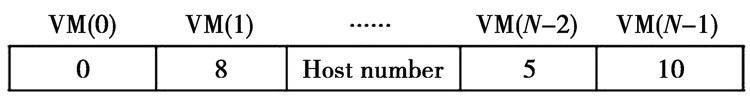图 2 个体编码示意图 Fig.2 Individual coding

3.2 适应度值评价与Pareto集合更新

 $\Delta {F_{{\rm{ power }}}}(indiv) = \sum\limits_{i = 0}^m {{f_{{\rm{ power }}}}} ({h_i} + v{m_{{\rm{ mig }}}}) - {f_{{\rm{ power }}}}({h_i})$ (14)
 $\Delta {F_{{\rm{ slatah }}}}({\rm{ }}indiv{\rm{ }}) = \sum\limits_{i = 0}^m {{f_{{\rm{ slatah }}}}} ({h_i} + v{m_{{\rm{ mig }}}}) - {f_{{\rm{ slatah }}}}({h_i})$ (15)
 $\begin{array}{*{20}{c}} {\Delta {F_{{\rm{ utilization }}}}({\rm{ }}indiv{\rm{ }}) = \sum\limits_{i = 0}^m {{f_{{\rm{ utilization }}}}} ({h_i} + v{m_{mig}}) - }\\ {{f_{{\rm{ utilization }}}}({h_i})} \end{array}$ (16)

(14)—(16)式分别估算了将待迁移的虚拟机按照个体进行放置后数据中心能耗增量、服务等级协议违例率增量与资源利用率增量。ΔFpower(indiv)的值越小，表示将虚拟机按照个体indiv放置后，数据中心能耗增量越小。由于对于不同个体数据中心的初始能耗相同，故而ΔFpower(indiv)越小，数据中心能耗越小。同理，ΔFslatah(indiv)值越小数据中心SLATAH值就越小。ΔFutilization(indiv)的值越大数据中心的资源利用率值越高。结合Pareto支配理念与(14)—(16)式，个体之间支配情况判断如下。

 $\begin{array}{*{20}{l}} {\Delta {F_{{\rm{ power }}}}({\rm{ }}indi{v_1}) \le \Delta {F_{{\rm{ power }}}}({\rm{ }}indi{v_2}) \wedge \Delta {F_{{\rm{ slatah }}}}({\rm{ }}indi{v_1}) \le }\\ {\Delta {F_{{\rm{ slatah }}}}({\rm{ }}indi{v_2}) \wedge \Delta {F_{{\rm{ utilization }}}}({\rm{ }}indi{v_1}) \ge }\\ {\Delta {F_{{\rm{ utilization }}}}({\rm{ }}indi{v_2})} \end{array}$
 $\begin{array}{*{20}{l}} {\Delta {F_{{\rm{ power }}}}({\rm{ }}indi{v_1}) < \Delta {F_{{\rm{ power }}}}({\rm{ }}indi{v_2}) \vee \Delta {F_{{\rm{ power }}}}({\rm{ }}indi{v_1}) < }\\ {\Delta {F_{{\rm{ slatah }}}}({\rm{ }}indi{v_2}) \vee \Delta {F_{{\rm{ utilization }}}}({\rm{ }}indi{v_1}) > }\\ {\Delta {F_{{\rm{ utilization }}}}({\rm{ }}indi{v_2}{\rm{ ) }}} \end{array}$

1) 如果$\exists$Pareto_indivPareto_Set，使得Search_indiv>Pareto_indiv成立, 则有Pareto_indiv=Search_indiv

2) 如果∀Pareto_indivPareto_Set，使得Search_indivPareto_indiv互不支配，则Pareto_Set=Pareto_Set∪{Search_indiv}。

3) 如果$\exists$Pareto_indivPareto_Set，使得Pareto_indiv>Search_indiv成立，则Search_indiv不是Pareto解。

3.3 多资源约束的局部搜索策略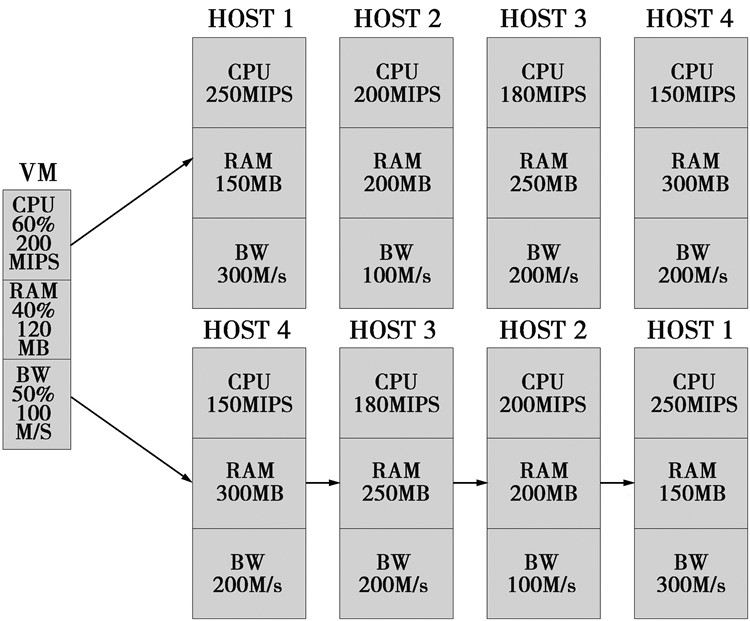图 3 局部搜索示意图 Fig.3 Local search

for (gene in individual)

Begin

vm=vmlist.get(gene.id)

将hostlist按照主要资源降序排列

For (host inhostlist)

Begin

If (虚拟机vm能够放置在物理主机host) then

Begin

将NewIndividual上vm对应的物理主机设置为host

Break

End If

End For

End For

If (NewIndiv==null) then NewIndiv=individual

3.4 交叉变异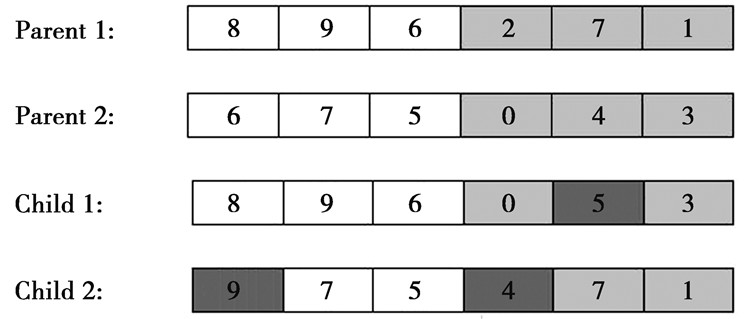图 4 个体交叉变异 Fig.4 Individual cross and variation

Parent 1与Parent2经过交叉、变异操作得到Child 1与Child 2。Child 1中有一位基因发生了变异。Child 2中有2位基因发生了变异。将经过变异的子代个体插入种群，同时移除其父代个体，生成新种群。

3.5 算法复杂度分析

MAVMP对局部搜索过程改进后，其局部搜索部分的时间复杂度由维护物理主机序列和按序搜索O(Q) 2部分组成。一次局部搜索中最多有2台物理主机受到影响，将2台物理主机重新插入有序物理主机序列的时间复杂度为O(logM)。由于分类后的虚拟机按照有序的物理主机序列能够快速找到合适物理主机，按需搜索所用时间远远小于物理主机维护时间，即Q < < logM，因此，MAVMP一次迭代的时间复杂度可以表示为O(XNlogM)，小于传统Memetic算法时间复杂度O(XNM)。由此可知，MAVMP方法能有效提高Memetic算法的时间效率。

4 实验设置 4.1 实验环境表 1 物理主机配置表 Tab.1 Physical machine instances表 2 虚拟机配置表 Tab.2 Virtual machine instances

CloudSim仿真平台的运行环境对算法的时间开销有着重要影响，为了公平对比各个算法的时效性，本文中所有仿真实验均在相同环境下进行，下面将对仿真平台所运行的环境进行具体介绍。实验所用机型为DELL inspiron 15-5577笔记本电脑，操作系统为WINDOWS 10，CPU型号为Intel Core i7-7700HQ 2.8 GHz，内存为8 GB。

4.2 工作负载表 3 BitBrains的工作负载特征 Tab.3 Properties of BitBrains trace
4.3 评价指标

SLATAH指由于活动物理主机在运行时资源过载导致的违反服务等级协议，定义如(4)式。PDM代表由于虚拟机迁移导致的服务质量下降，定义式为

 $PDM = \frac{1}{m}\sum\limits_{j = 1}^m {\frac{{C_j^d}}{{C_j^r}}}$ (17)

(17) 式中：Cjd代表虚拟机vmj由于迁移导致的未被满足的CPU资源请求量；Cjr代表虚拟机vmj的CPU资源总量；m代表虚拟机数量。

SLAV是用来评价单日内数据中心服务质量的综合指标，定义如(18)式。SLAV的值受当日数据中心SLATAHPDM的综合影响。当日数据中心的SLATAHPDM值越低则综合值SLAV越小，数据中心服务质量越高。

 $SLAV = SLATAH \times PDM$ (18)

EC代表数据中心一天的能源消耗。定义如(1)式。根据综合数据中心对低能耗和高服务质量的要求，定义服务质量和能耗综合评价指标ESV，如(19)式。ESV的值越低意味着当日数据中心的能耗和服务质量越平衡。

 $ESV = EC \times SLAV$ (19)

5 实验结果及分析

5.1 有效性表 4 BitBrains数据集测试结果 Tab.4 Simulation results using BitBrains trace

EC指标能够反映出不同方法对数据中心能耗的影响。通过表 4可以发现MAVMP方法的EC值最低，EOPVMP与PABFD方法略高于MAVMP方法，这说明了MAVMP方法能够有效优化数据中心能耗。由于VMPACS方法在放置虚拟机时缺乏对数据中心的能耗考虑，因此，VMPACS方法的EC值最高。

SLAV值代表了数据中心的服务质量(QoS)，SLAV值越低数据中心的QoS越能得到保障。根据表 4可以发现MAVMP方法的SLAV值最小。这说明在5种不同的虚拟机放置方法中，采用MAVMP方法能够充分保障数据中心的QoS。FFD和PABFD方法的SLAV值较高，这是因为FFD与PABFD方法在进行虚拟机放置时忽视了虚拟机请求的变化。EOPVMP和ACS方法的SLAV值虽然高于MAVMP方法，但其对改善服务质量也有一定作用。

SLAV值是SLATAH值与PDM值的综合体现。为了深入分析不同方法对数据中心服务质量的影响，本文通过图 5图 6对比了5种虚拟机放置方法的SLATAH值与PDM值。因为MAVMP方法将最小化运行时服务等级协议违例时间作为优化目标，所以根据图 5可以看出，MAVMP方法的SLATAH值最小。VMPACS方法的SLATAH值与MAVMP相近。根据图 6可以发现在5种放置方法中，MAVMP方法的PDM值最小。这是因为MAVMP通过合理的虚拟机放置稳定了物理负载，从根本上减少了过载物理主机数量。过载物理主机的减少使得虚拟机迁移量下降，因此，MAVMP方法比其他4种方法能够更有效减少虚拟机迁移对数据中心QoS的影响。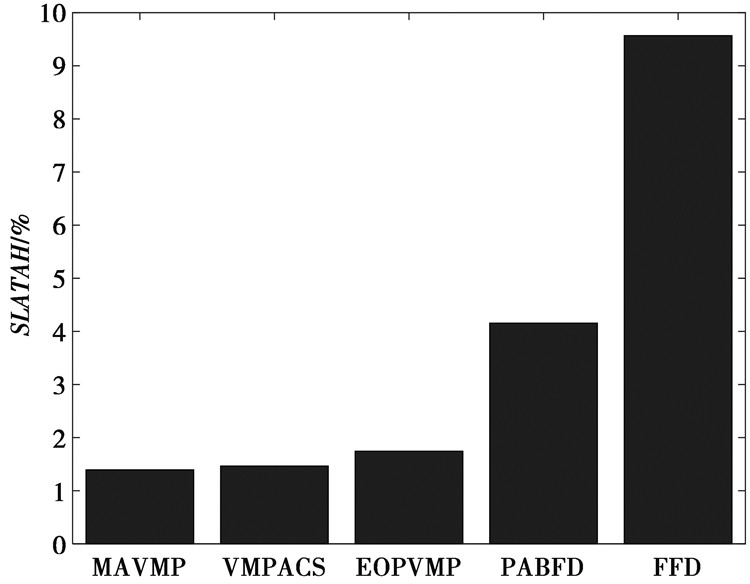图 5 SLATAH指标对比 Fig.5 Comparison of SLATAH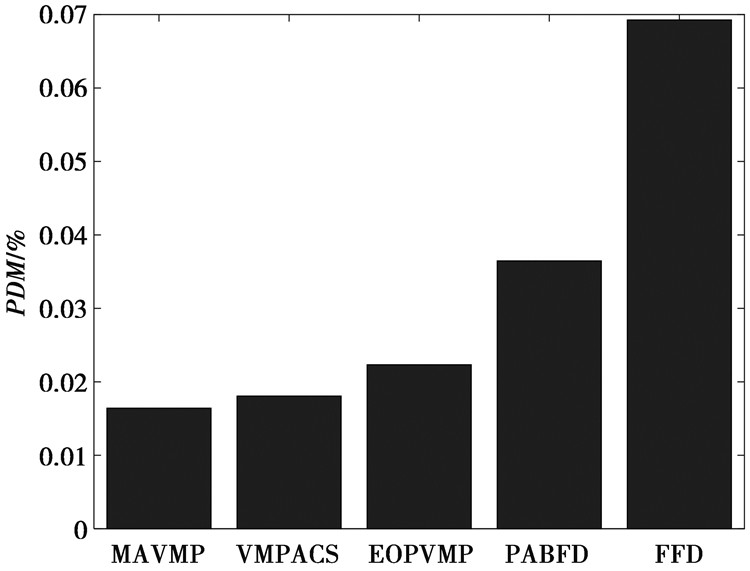图 6 PDM指标对比 Fig.6 Comparison of PDM

ESV是体现数据中心能耗与服务质量的综合指标。通过表 4可以看出，MAVMP方法的ESV值在5种方法中最低。这说明了MAVMAP方法能够利用Pareto支配理念在降低能耗和提高服务质量2个目标中找到折衷解，同时进一步说明了MAVMP方法在虚拟机放置过程中的有效性。

5.2 高效性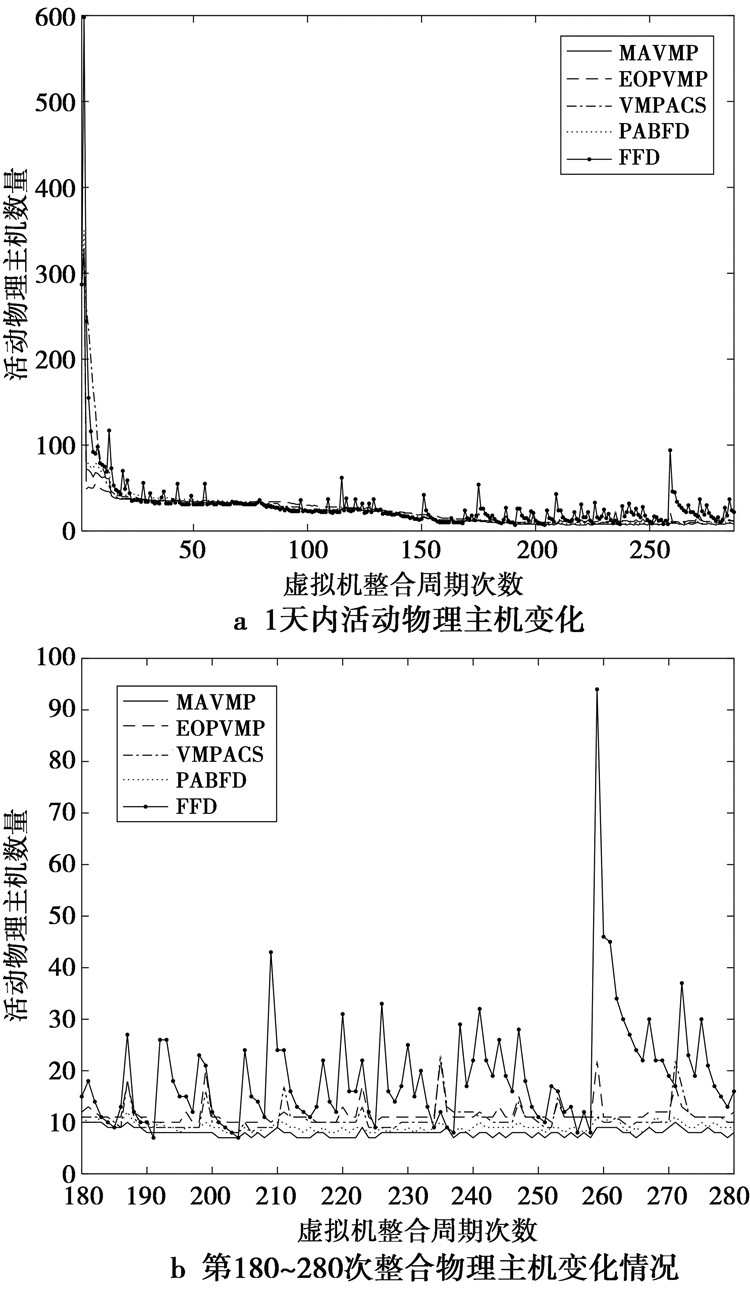图 7 活动物理主机数量 Fig.7 Number of running physical host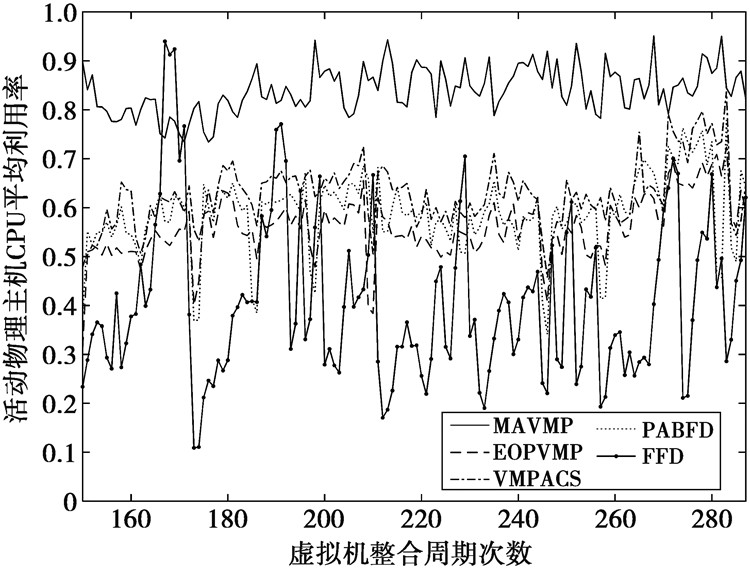图 8 物理主机CPU资源利用率 Fig.8 CPU utilization of running physical host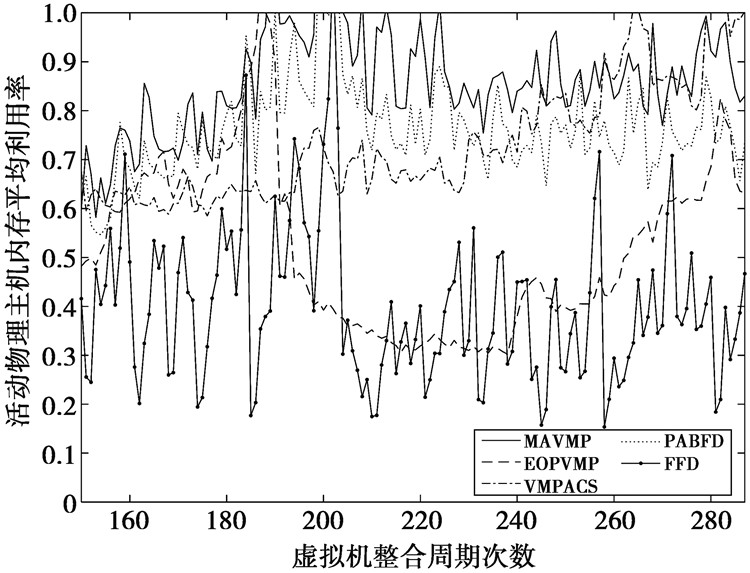图 9 物理主机内存资源利用率 Fig.9 RAM utilization of running physical host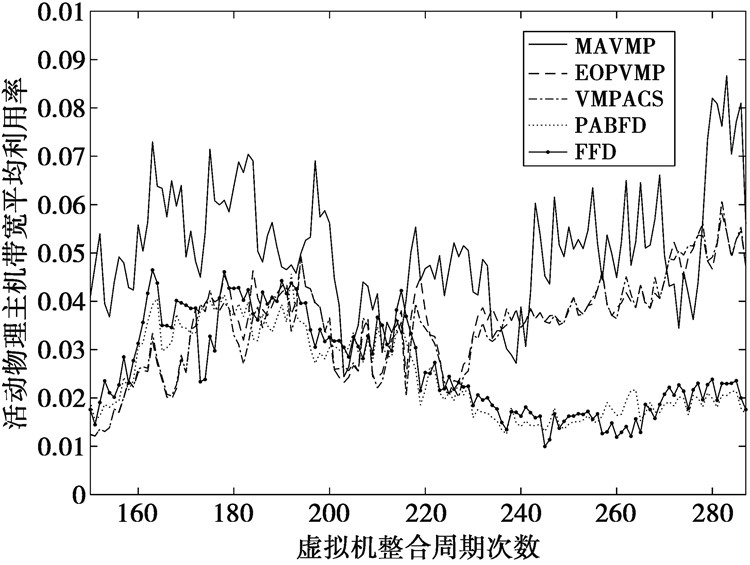图 10 物理主机带宽资源利用 Fig.10 BW utilization of running physical host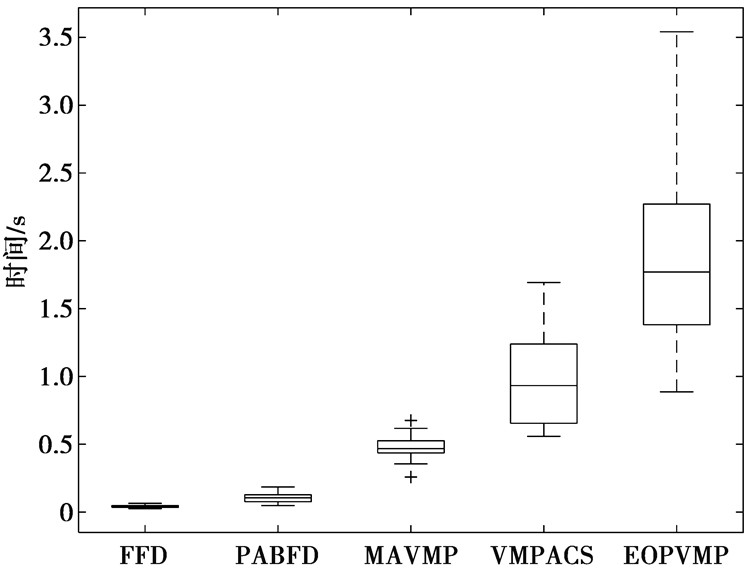图 11 时间开销 Fig.11 Time cost

6 总结